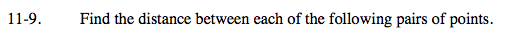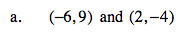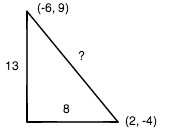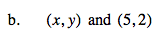Home > A2C > Chapter 11 > Lesson 11.1.1 > Problem11-9

11-9.
1. Find the distance between each of the following pairs of points. Homework Help ✎

1. (−6, 9) and (2, −4)

2. (x, y) and (5, 2)See part (a).

$\sqrt{(x - 5)^2+(y - 2)^2}$